## ML Aggarwal Class 6 Solutions for ICSE Maths Chapter 7 Decimals Check Your Progress

Question 1.
Convert the following decimal numbers into fractions (in lowest terms).
(i) 6.015
(ii) 0.876
(iii) 23.375
Solution:
(i) 6.015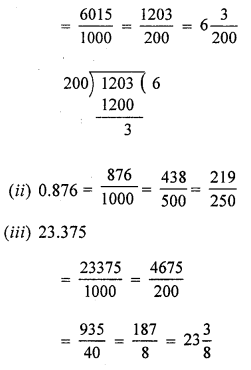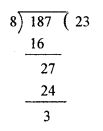Question 2.
Write the following fractions as decimals numbers: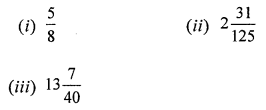Solution: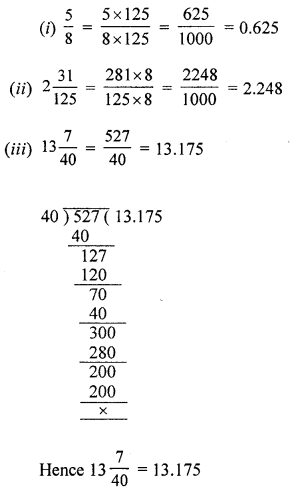Question 3.
Arrange the following decimal numbers in ascending order:
(i) 123.8, 74.205, 74.209, 7.4209
(ii) 85.01, 85.1, 85.001, 85.103
Solution:
(i) 7.4209, 74.205, 74.209, 123.8
(ii) 85.001, 85.01, 85.1, 85.103

Question 4.
Arrange the following decimal numbers in desending order:
(i) 6.45, 4.65, 6.405, 64.5, 6.54
(ii) 73.5, 35.7, 7.35, 7.53, 7.035
Solution:
(i) 64.5 > 6.54 > 6.45 > 6.405 > 4.65
(ii) 73.5 > 35.7 > 7.53 > 7.35 > 7.035

Question 5.
If the school bags of Garima and Nakul weigh 5.2 kg and 4.832 kg respectively, find
(i) the total weight.
(ii) the difference in weight of the bags.
Solution:
Weight of Garima’s bag = 5.2 kg.
Weight of Nakul’s bag = 4.832 kg.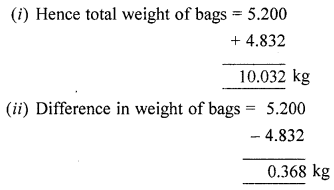Question 6.
Evaluate the following:
(i) 31.42 – 17.853 -6.43
(ii) 13.01 – 5.428 – 3.703 + 2.99.
Solution:
(i) 31.42 – 17.853 – 6.43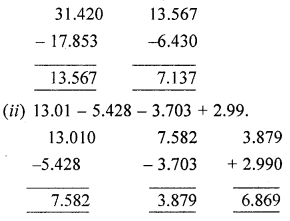Question 7.
By how much does the sum of 15.453 and 31.647 exceed the sum of 18.47 and 19.506?
Solution: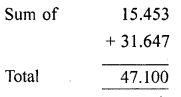Question 8.
Namita travels 20 km 50 m every day. Out of this she travels 10 km 200 m by bus and the rest by auto. How much distance does she travel by auto?
Solution:
Distance travelled everyday
= 20 km 50 m = 20 km + 50 m
= 20 km + $$\frac{50}{1000}$$ km
= 20 km + 0.050 km [∵ $$\frac{1}{1000}$$km = 0.001 km]
= (20 + 0.050) km = 20.050 km
Distance travelled by bus
= 10 km 200 m = 10 km + 200 m
= 10 km + $$\frac{200}{1000}$$ km
= 10 km + 0.200 km [∵ $$\frac{1}{1000}$$ km = 0.001 km]
= (10 + 0.200) km = 10.200 km
∴ Distance travelled by auto
= 20.050 km – 10.200 km = 9.850 km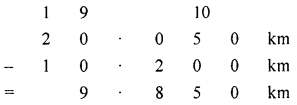Question 9.
Ravi purchased 5 kg 400 g rice, 2 kg 20 g sugar and 10 kg 850 g flour (aata). Find the total weight of his purchases.
Solution:
Weight of rice purchased
= 5 kg 400 g = 5 kg + 400 g
= 5 kg + $$\frac{400}{1000}$$ kg [∵ 1 g = $$\frac{1}{1000}$$ kg]
= 5 kg + 0.400 kg
= (5 + 0.400) kg = 5.400 kg
Weight of sugar purchased
= 2 kg 20 g = 2 kg + 20 g
= 2 kg = $$\frac{20}{1000}$$ kg [∵ 1 g = $$\frac{1}{1000}$$ kg]
= 2 kg + 0.020 kg = 2.020 kg
Weight of flour purchased
= 10 kg 850 g = 10 kg + 850 g
= 10 kg + $$\frac{850}{1000}$$ kg [∵ 1 g = $$\frac{1}{1000}$$ kg]
= 10 kg + 0.850 kg
= (10 + 0.850) kg = 10.850 kg
∴ Total weight of his purchases is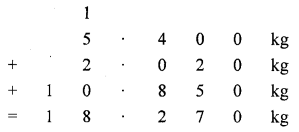Question 10.
1 kg of pure milk contains 0.263 kg of fat. How much fat is there 15.5 kg of milk?
Solution:
1 kg of pure milk contains fat = 0.263 kg
∴ 15.5 kg of milk contain fatQuestion 11.
The product of two numbers is 15.275. If one number is 4.7, find the other.
Solution:
The product of two number = 15.275
One number = 4.7
∴ Then other is = $$\frac{15.275}{4.7}=3.25$$